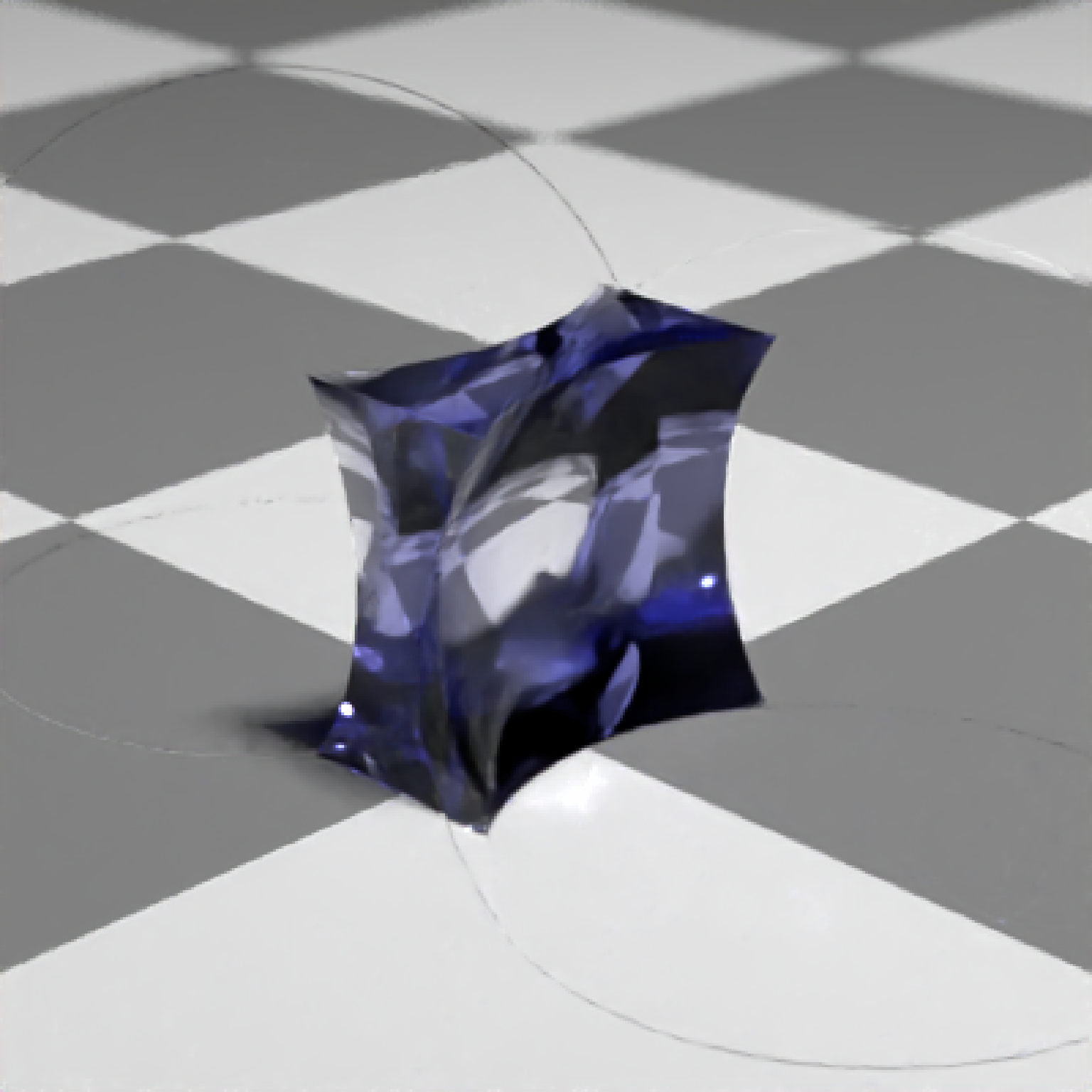Dielectric (glass) Material

dielectric(
color = "white",
refraction = 1.5,
attenuation = c(0, 0, 0),
attenuation_intensity = 1,
priority = 0,
importance_sample = FALSE,
bump_texture = NA,
bump_intensity = 1
)

## Arguments

color

Default white. The color of the surface. Can be either a hexadecimal code, R color string, or a numeric rgb vector listing three intensities between 0 and 1.

refraction

Default 1.5. The index of refraction.

attenuation

Default c(0,0,0). The Beer-Lambert color-channel specific exponential attenuation through the material. Higher numbers will result in less of that color making it through the material. If a character string is provided (either as a named R color or a hex string), this will be converted to a length-3 vector equal to one minus the RGB color vector, which should approximate the color being passed. Note: This assumes the object has a closed surface.

attenuation_intensity

Default 1. Changes the attenuation by a multiplicative factor. Values lower than one will make the dielectric more transparent, while values greater than one will make the glass more opaque.

priority

Default 0. When two dielectric materials overlap, the one with the lower priority value is used for intersection. NOTE: If the camera is placed inside a dielectric object, its priority value will not be taken into account when determining hits to other objects also inside the object.

importance_sample

Default FALSE. If TRUE, the object will be sampled explicitly during the rendering process. If the object is particularly important in contributing to the light paths in the image (e.g. light sources, refracting glass ball with caustics, metal objects concentrating light), this will help with the convergence of the image.

bump_texture

Default NA. A matrix, array, or filename (specifying a greyscale image) to be used to specify a bump map for the surface.

bump_intensity

Default 1. Intensity of the bump map. High values may lead to unphysical results.

## Value

Single row of a tibble describing the dielectric material.

## Examples

#Generate a checkered ground
scene = generate_ground(depth=-0.5, material = diffuse(checkercolor="grey30",checkerperiod=2))
if(run_documentation()) {
render_scene(scene,parallel=TRUE)
}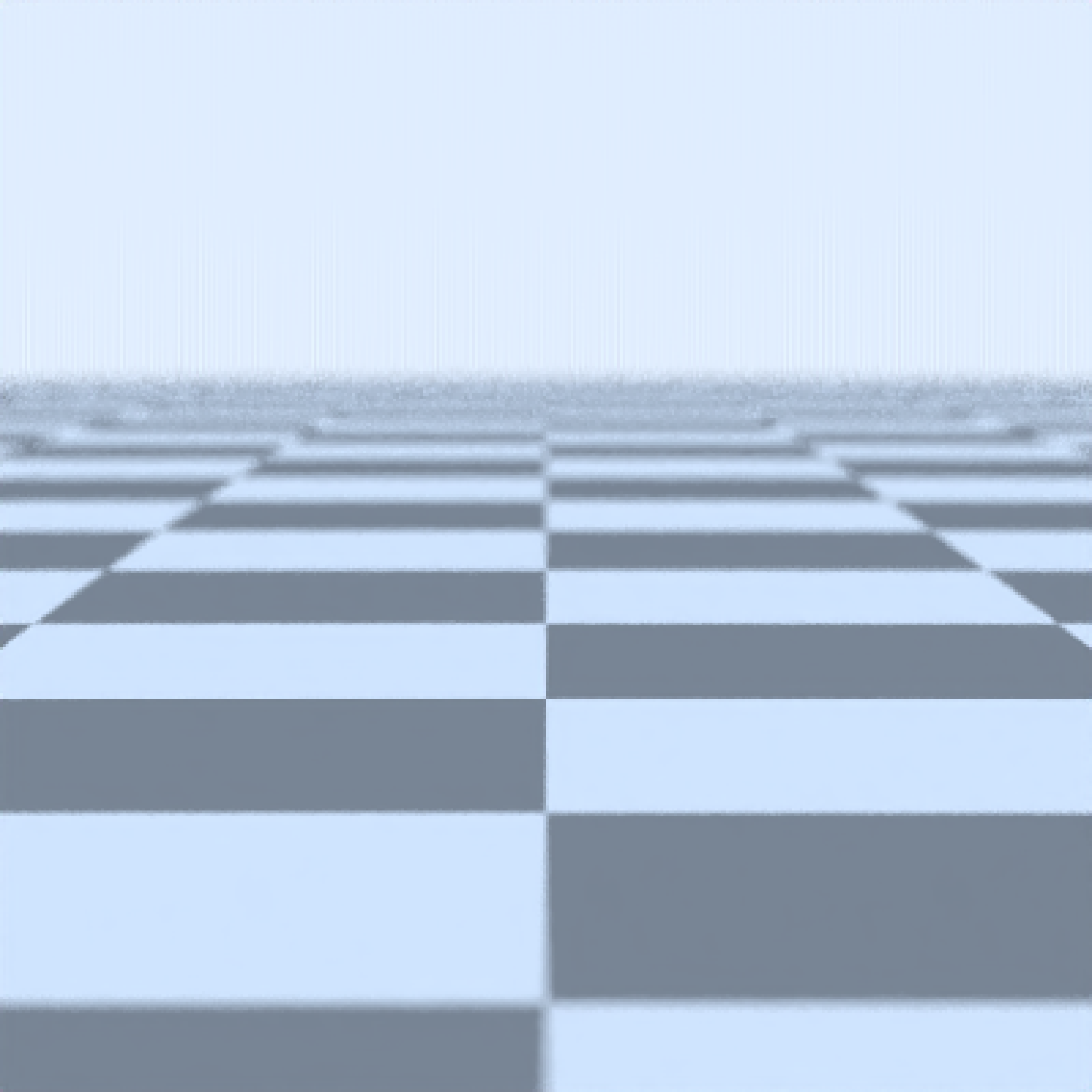if(run_documentation()) {
scene %>%
render_scene(parallel=TRUE,samples=128)
}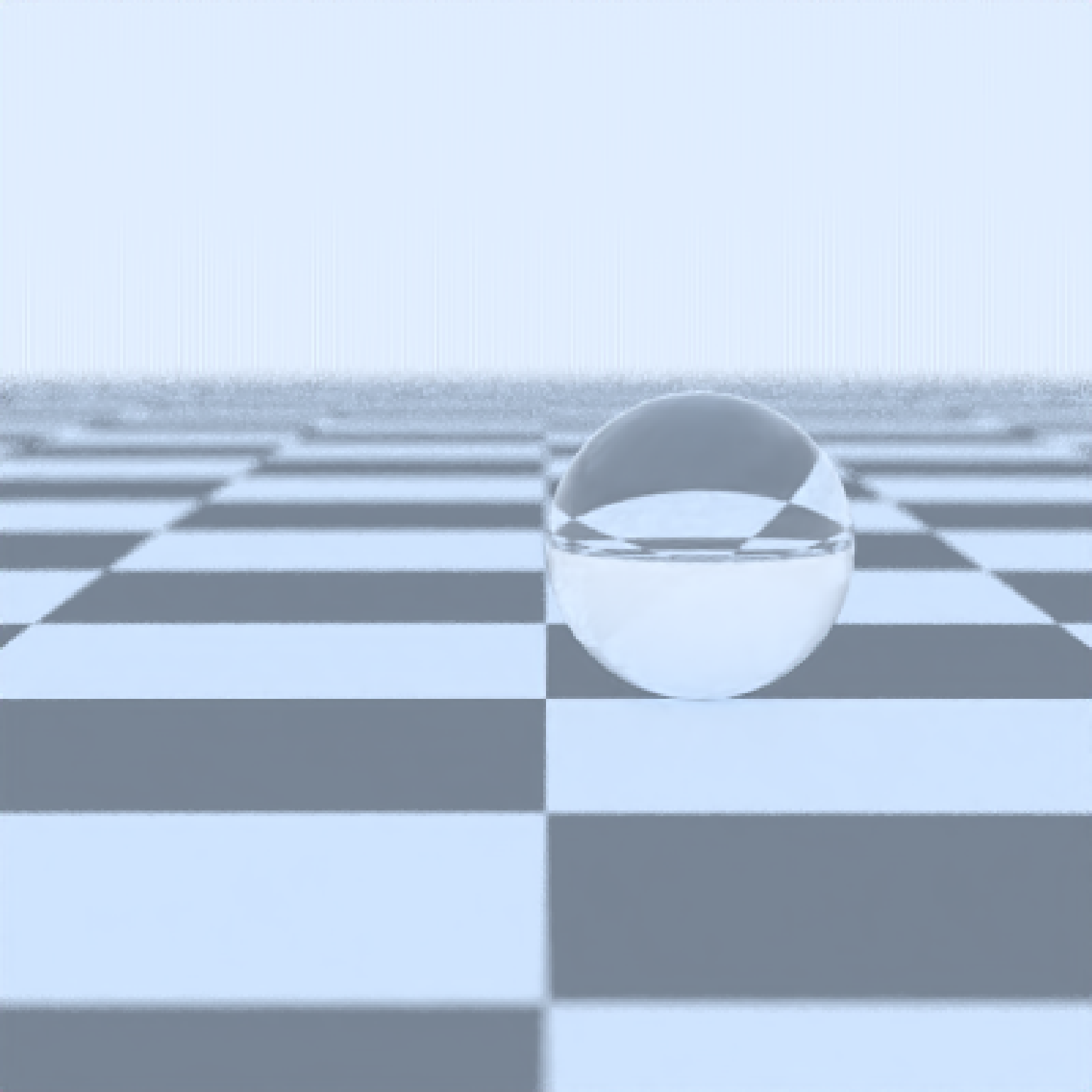#Add a rotated colored glass cube
if(run_documentation()) {
scene %>%
render_scene(parallel=TRUE,samples=128)
}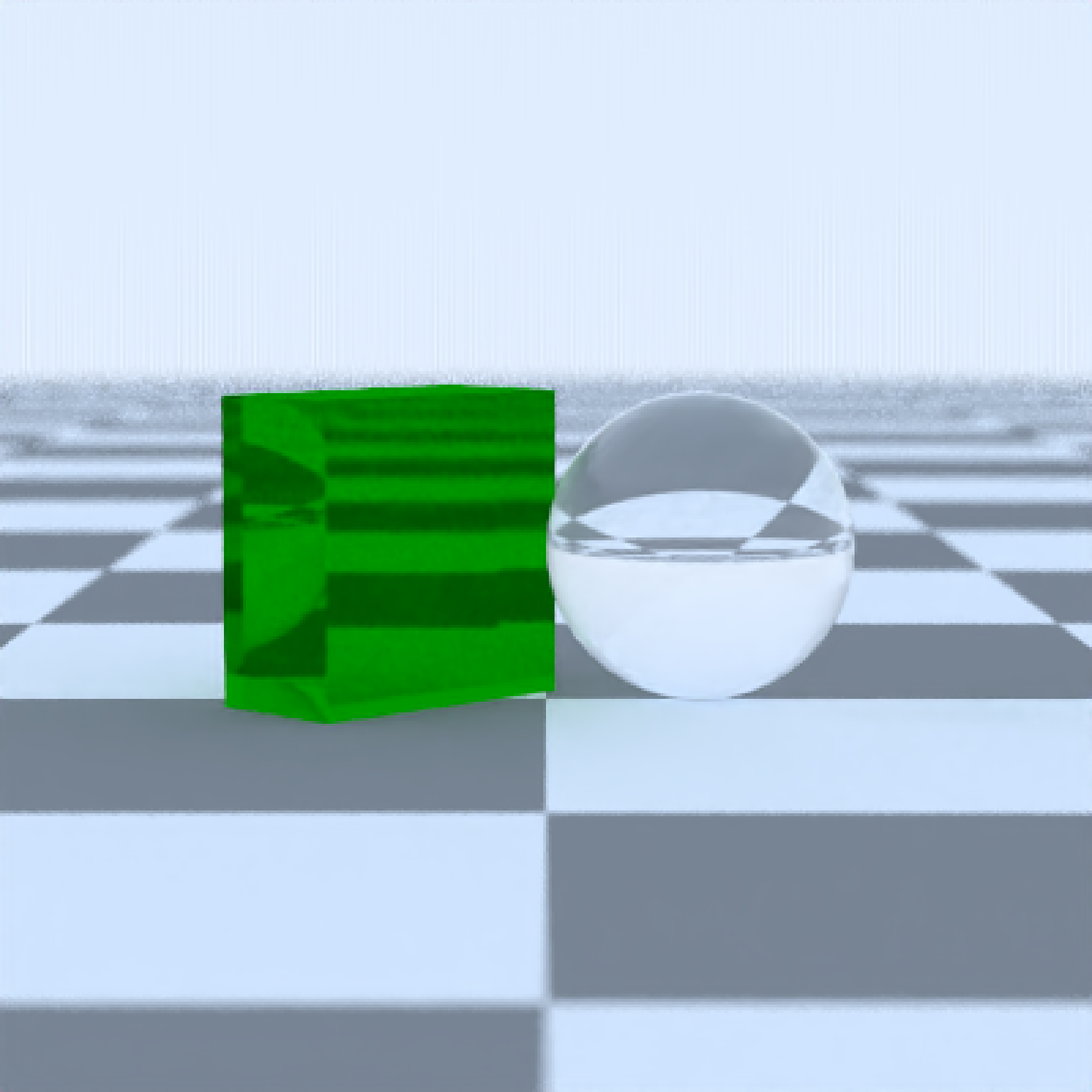#Add an area light behind and at an angle and turn off the ambient lighting
if(run_documentation()) {
scene %>%
material=light(intensity=15),
angle=c(0,-90,45), order_rotation = c(3,2,1))) %>%
render_scene(parallel=TRUE,aperture=0, ambient_light=FALSE,samples=1000)
}
#> Warning: "sobol_blue" sample method only valid for samples than or equal to 256--switching to sample_method = "sobol"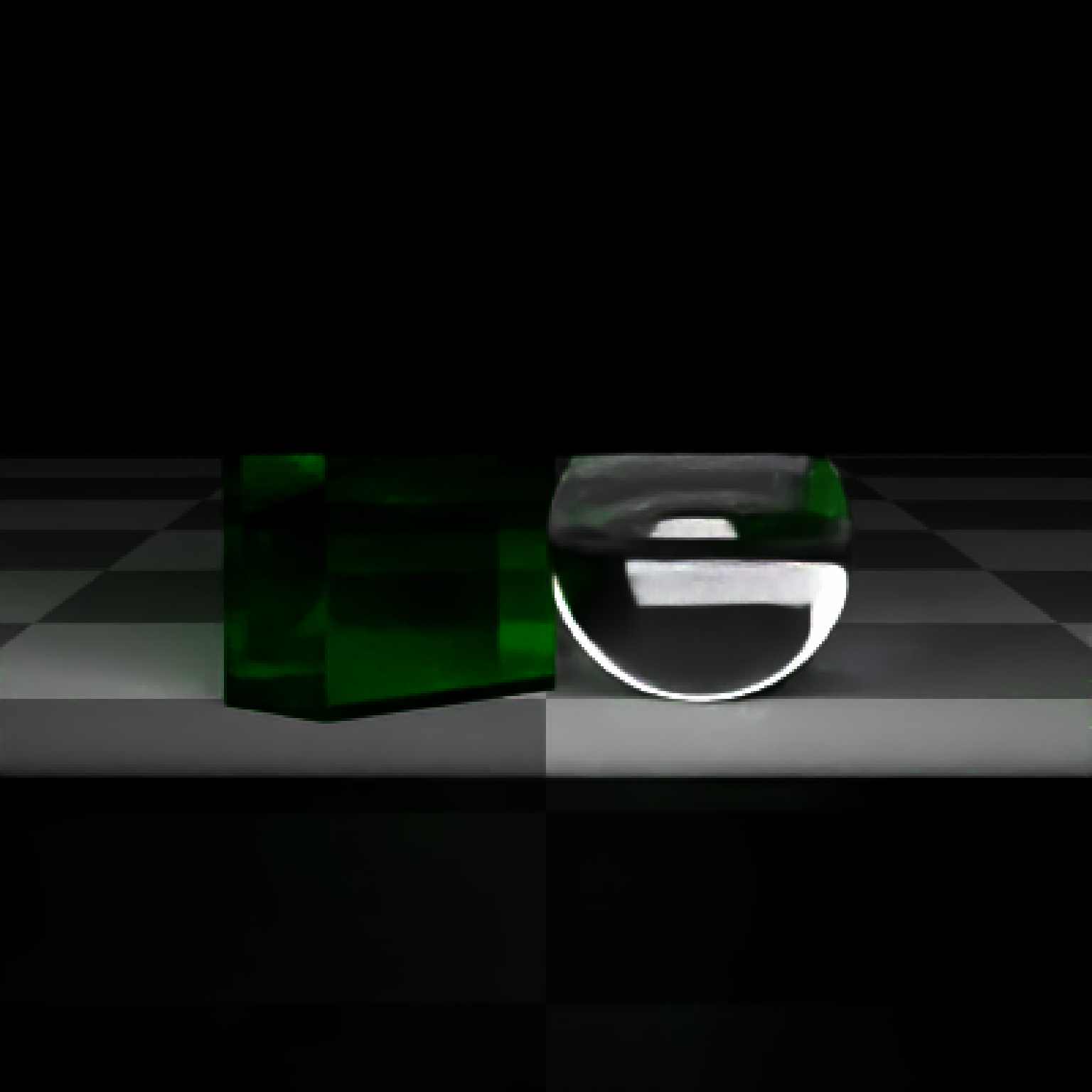#Color glass using Beer-Lambert attenuation, which attenuates light on a per-channel
#basis as it travels through the material. This effect is what gives some types of glass
#a green glow at the edges. We will get this effect by setting a lower attenuation value
#for the green (second) channel in the dielectric attenuation argument.
if(run_documentation()) {
generate_ground(depth=-0.5,material=diffuse(checkercolor="grey30",checkerperiod=2)) %>%
material=dielectric(attenuation=c(1.2,0.2,1.2)),angle=c(45,110,0))) %>%
render_scene(parallel=TRUE, samples = 1000)
}
#> Warning: "sobol_blue" sample method only valid for samples than or equal to 256--switching to sample_method = "sobol"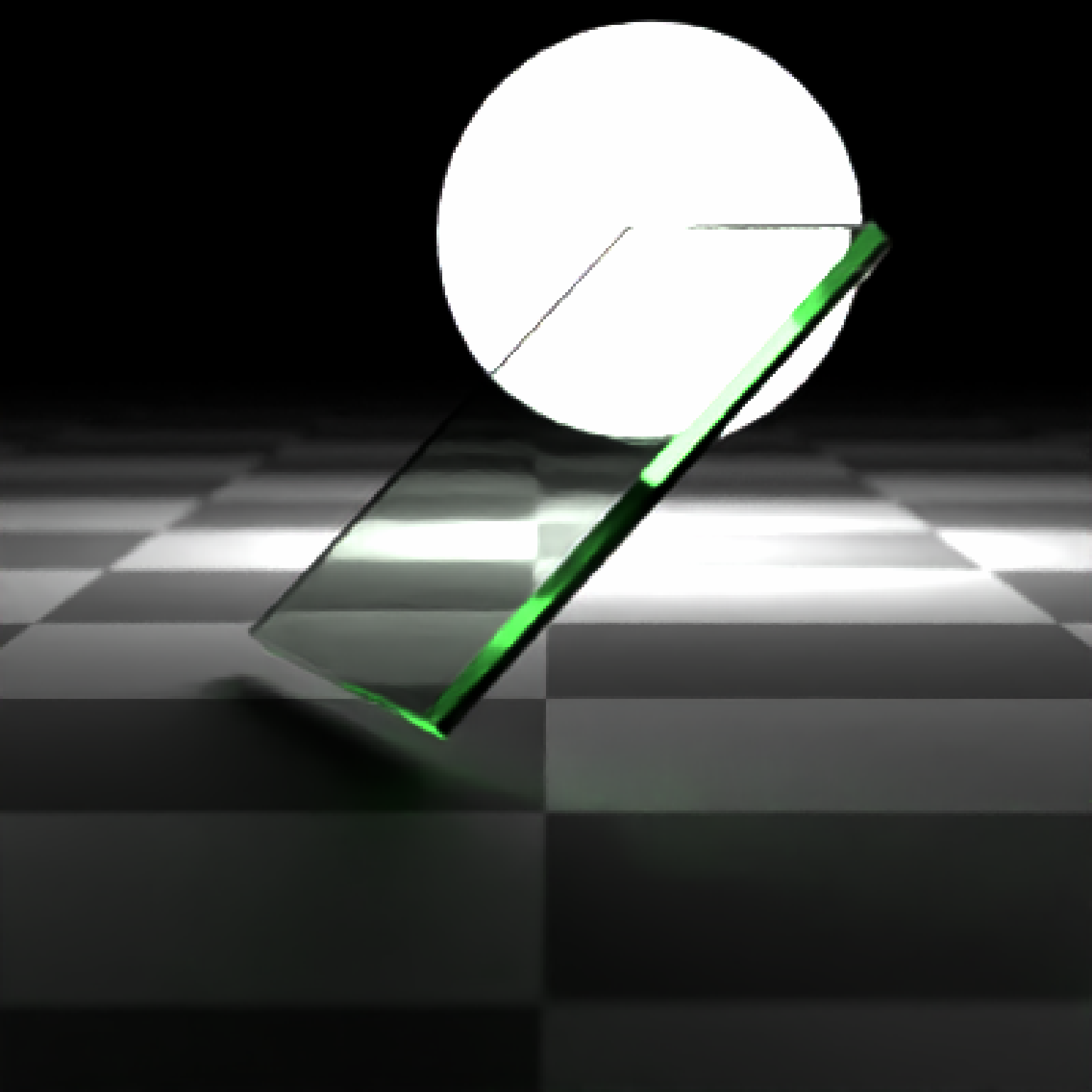#If you have overlapping dielectrics, the priority value can help disambiguate what
#object wins. Here, I place a bubble inside a cube by setting a lower priority value and
#making the inner sphere have a index of refraction of 1. I also place spheres at the corners.
if(run_documentation()) {
generate_ground(depth=-0.51,material=diffuse(checkercolor="grey30",checkerperiod=2)) %>%
add_object(cube(material = dielectric(priority=2, attenuation = c(10,3,10)))) %>%
material = dielectric(priority=0,attenuation = c(10,3,10) ))) %>%
material = dielectric(priority=0,attenuation = c(10,3,10)))) %>%
render_scene(parallel=TRUE, samples = 128,lookfrom=c(5,1,5))
}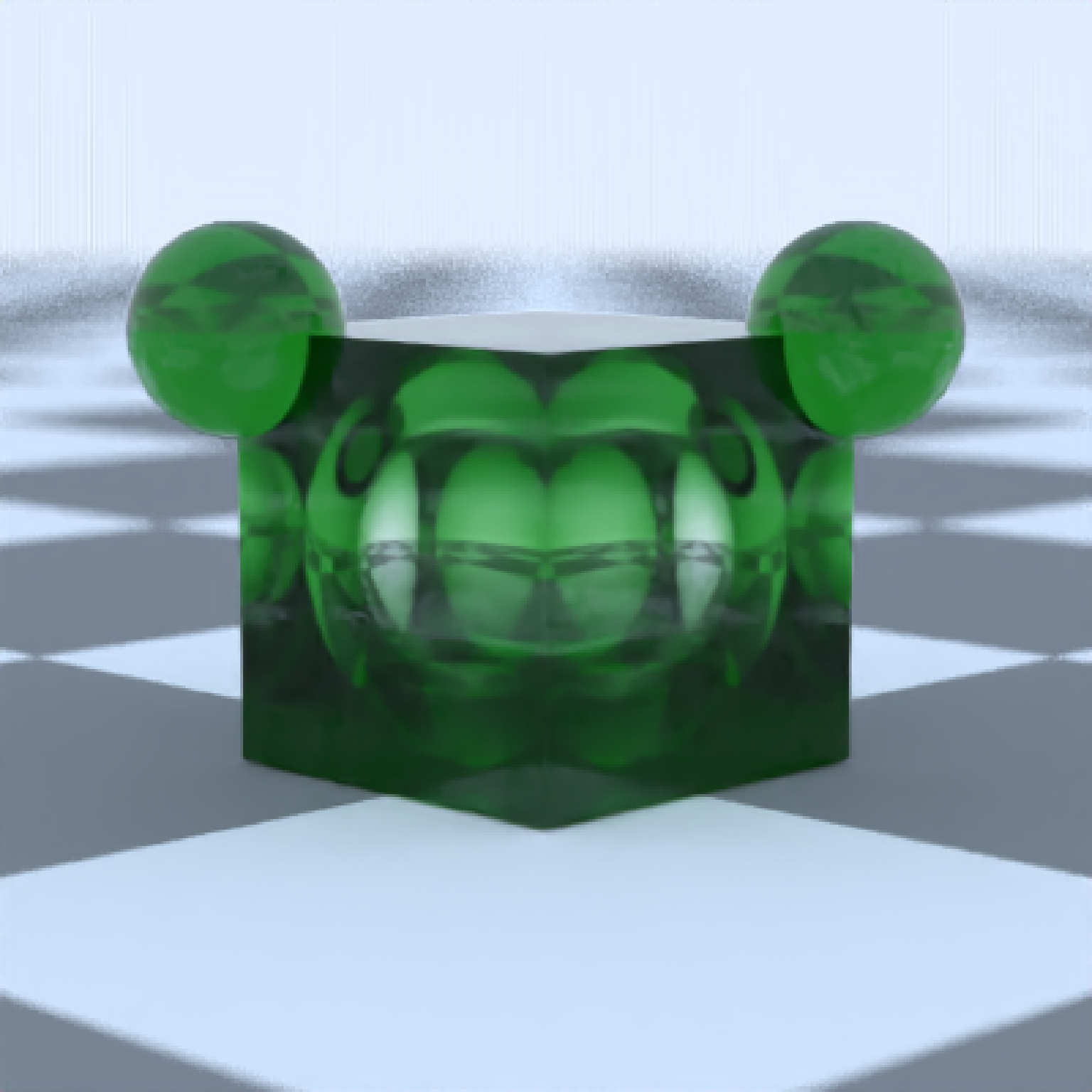# We can also use this as a basic Constructive Solid Geometry interface by setting
# the index of refraction equal to empty space, 1. This will subtract out those regions.
# Here I make a concave lens by subtracting two spheres from a cube.
if(run_documentation()) {
generate_ground(depth=-0.51,material=diffuse(checkercolor="grey30",checkerperiod=2,sigma=90)) %>%
add_object(cube(material = dielectric(attenuation = c(3,3,1),priority=1))) %>%